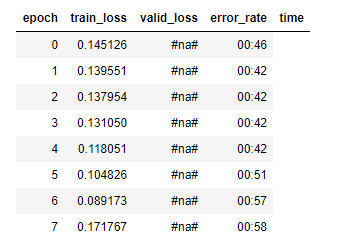# 📝 Deep Learning Lesson 2 Notes

Thanks a lot, i spent like 30 mins trying to figure out why i couldnt download. Appreciate it!

Hi all,

I have one quick question regarding the `update()` function. Been trying to wrap my head around this concept.

Question:

How do we know that if we move the whole thing downwards, the loss goes up and vice versa?

Appreciate any insights. Thank you.

I believe the learned coefficients in the linear recession example will tend to (3,2.5).

So, I am using Mozilla Firefox, and it seems I can download the links using the code in the tutorial, but am I supposed to save the file in a particular directory? Or is there a different javascript for Firefox to download the links?

Hello. I know this reply is a bit late but it may still be of help to someone else. If i understand, you’re confused about why the loss goes up when the whole thing is moved downwards. Think of it this way, under normal conditions, when the gradient of a quadratic function is taken, it gives us the direction that increases the loss. Instead of increasing the loss, we desire the loss to be decreased so what we do is to take the negative of the gradient.

I saw a pretty good explanation here. https://medium.com/@aerinykim/why-do-we-subtract-the-slope-a-in-gradient-descent-73c7368644fa

I was confused because I didn’t realise that (1) he was referring to the gradient of the loss func and (2) I couldn’t visualize it until someone drew it out clearly

Hi all,

I’m having trouble after cleaning my images and training the model with the new databunch created from the new cleaned csv.
When getting the learn rate (learn.lr_find()), im getting #na# values instead of valid_lossess. It seems columns are displazed to the left as instead or error_rate im getting in that column the time.This is normal, `valid_loss` and `error_rate` are not calculated by the learning rate finder. It only checks for the loss on the test set, this is because our aim is to determine the learning rate using lr_find() and not to train the model. (that will be done later using `learn.fit_one_cycle()`)

Oh i thought that lr_find calculates the valid_loss and the error_rate for each lr it tries out.
Then why is the time column in the error_rate column?
Thanks!

I think you see the time values below error_rate because the error_rate value is just null and 0 characters long so the next value is displayed just after.

Hello.
Is Stochastic gradient descent technique same as Stochastic deep learning??